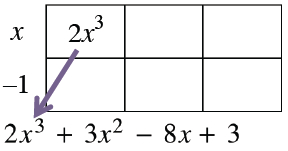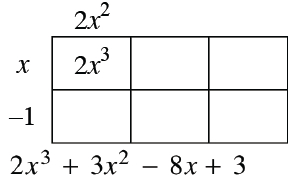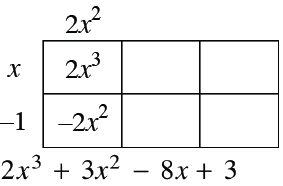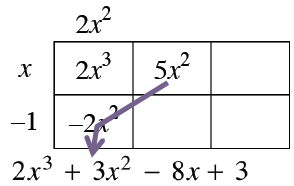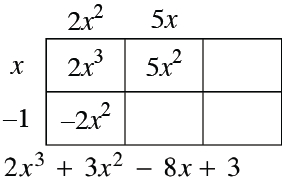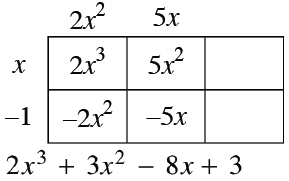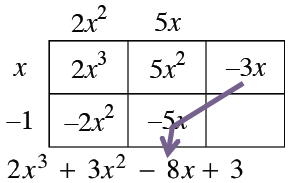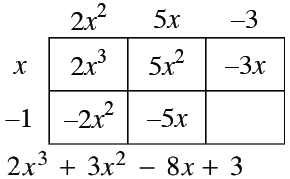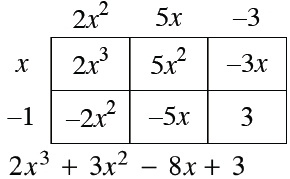### Home > INT3 > Chapter 8 > Lesson 8.3.1 > Problem8-93

8-93.

Now Carlos needs to solve $2x^{3} + 3x^{2} – 8x + 3 = 0$, but his calculator will still only create a graph with a standard viewing window. He sees that the graph of
$y = 2x^{3} + 3x^{2} – 8x + 3$ crosses the $x$‑axis at $x = 1$. What are all three solutions to $2x^{3} + 3x^{2} – 8x + 3 = 0$? Homework Help ✎

If $x = 1$, then $x − 1 = 0$.
So divide $2x^{3} + 3x^{2} − 8x + 3 = 0$ by $x − 1$.

Write the equation in factored form.
$\left(x − 1\right)\left(2x^{2} + 5x − 3\right) = 0$

Factor the quadratic expression and use the Zero Product Property to obtain the three solutions.